# Gestep Function In Excel

• Last Updated : 09 May, 2021

GESTEP is a function in Microsoft Excel that is used to check whether the given number is greater than the given threshold value(given step value). It returns 1 i.e.  True , if the number is greater than the step value and, It returns 0 i.e. False , if the number is lesser than the step value.

Formula of GESTEP function:

Attention reader! Don’t stop learning now. If you are an Excel beginner (or an intermediate) and want to learn Excel, Geeksforgeeks brings the perfect course for you to start, Diving Into Excel

`=GESTEP(number, [step])`

Here,

•  Number= given number that is to be tested.
• Step=defines the step size with which given number is compared. Step is completely optional. If step value is not given it is considered as 0

Example:

Lets suppose we have following numbers and steps, and we have to use the GESTEP function.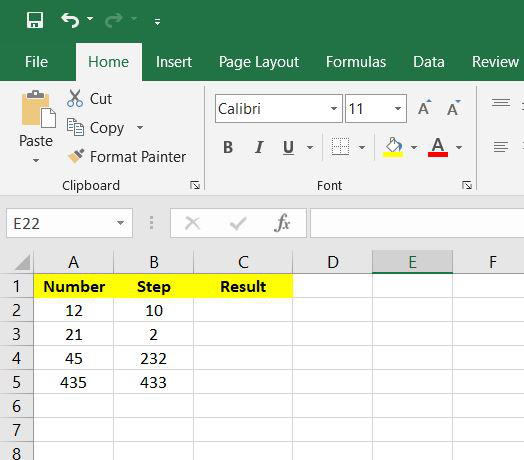Step 1: Now we will write =GESTEP(A2,B2), here A2 and B2 are the number and step values respectively. Now press enter.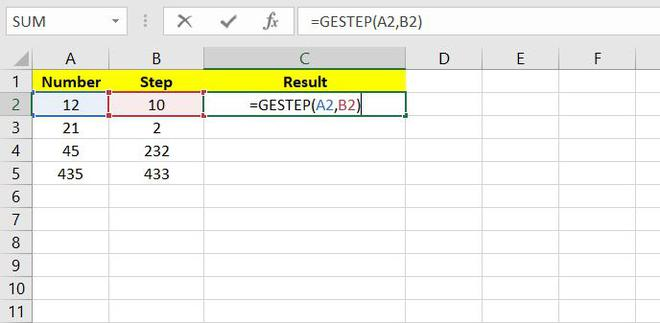Step 2: Now we can say that the Result value is 1 i.e. True . This is because the number value>Step value.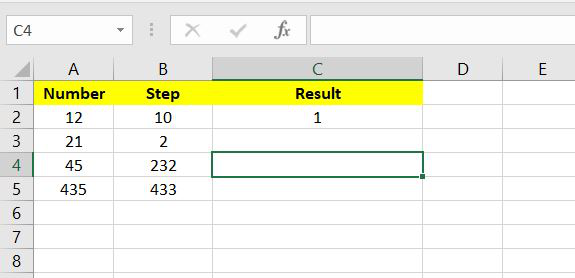Step 3: Now Drag and select from C2 to C5 and all the values for each row will be automatically calculated.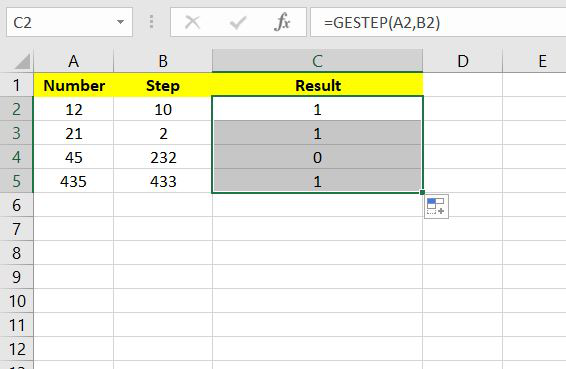Step 4: This is the final result of the given question.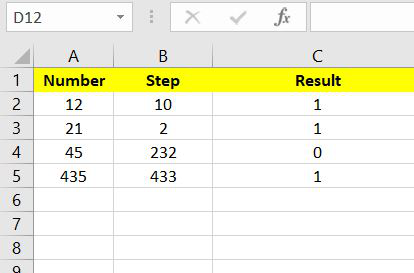### Common error:

#VALUE! =  This occurs if any of the values or both the values in the GESTEP function are non-numeric.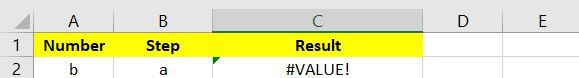My Personal Notes arrow_drop_up